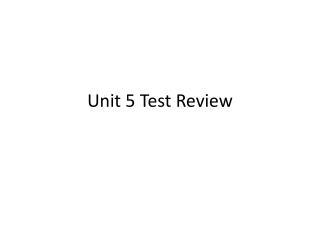Download PresentationUnit 5 Test ReviewUnit 5 Test Review - PowerPoint PPT Presentation

Download PresentationUnit 5 Test Review
An Image/Link below is provided (as is) to download presentation

Download Policy: Content on the Website is provided to you AS IS for your information and personal use and may not be sold / licensed / shared on other websites without getting consent from its author. While downloading, if for some reason you are not able to download a presentation, the publisher may have deleted the file from their server.

- - - - - - - - - - - - - - - - - - - - - - - - - - - E N D - - - - - - - - - - - - - - - - - - - - - - - - - - -
Presentation Transcript

1. Unit 5 Test Review

2. Which of the following is true for all parallelograms? • A. Opposite angles are congruent. • B. Diagonals are congruent. • C.Consecutive angles are complementary. • D. Diagonals are perpendicular.

3. Which of the following is true for all trapezoids? • A. Diagonals are congruent. • B. Diagonals are perpendicular. • C. Consecutive angles between parallel lines are complementary. • D. Consecutive angles between parallel lines are supplementary.

4. Determine which quadrilaterals always have perpendicular diagonals. • Squares • Rectangles • Rhombuses • Parallelograms • A. Only I. • B. Only I and II. • C. Only I and III. • D. I, II, III, and IV.

5. In any parallelogram ABCD, it is always true that the measures of ABC and CDA: DRAW A PICTURE • add up to 180 • are each 90 • are always congruent • are each less than 90

6. Given the following information about quadrilateral ABCD; sketch, label, and name a type of quadrilateral that meets these conditions. AC  BD AB // CD

7. Given rhombus ABCD. Use coordinate geometry to demonstrate that the diagonals of ABCD are perpendicular. A B D C

8. L K J Find the 4th vertex rectangle JKLM.

9. The slopes and lengths of 5 diagonals are given. Determine which two could be the diagonals of the same square.

10. The slopes and lengths of 5 diagonals are given. Determine which two could be the diagonals of the same square.

11. QRST is a parallelogram. Find QT.